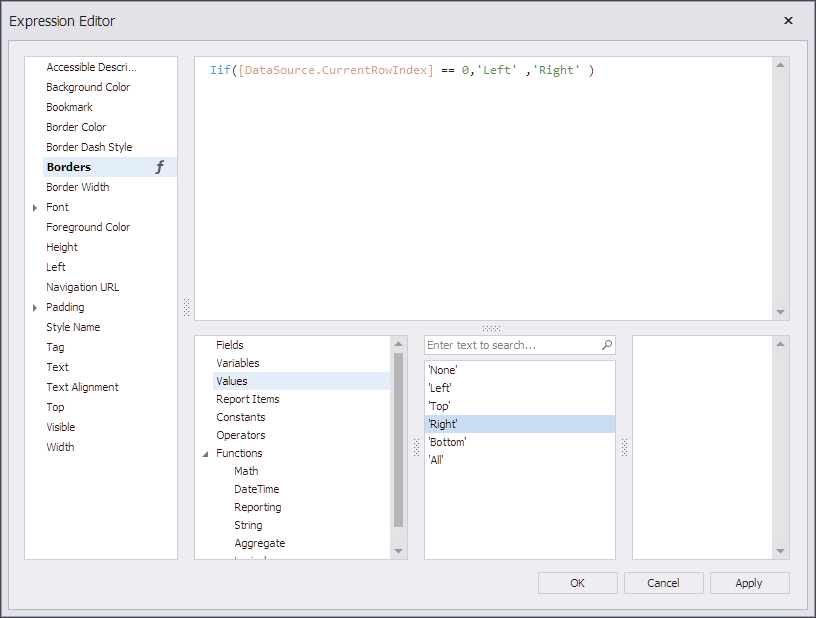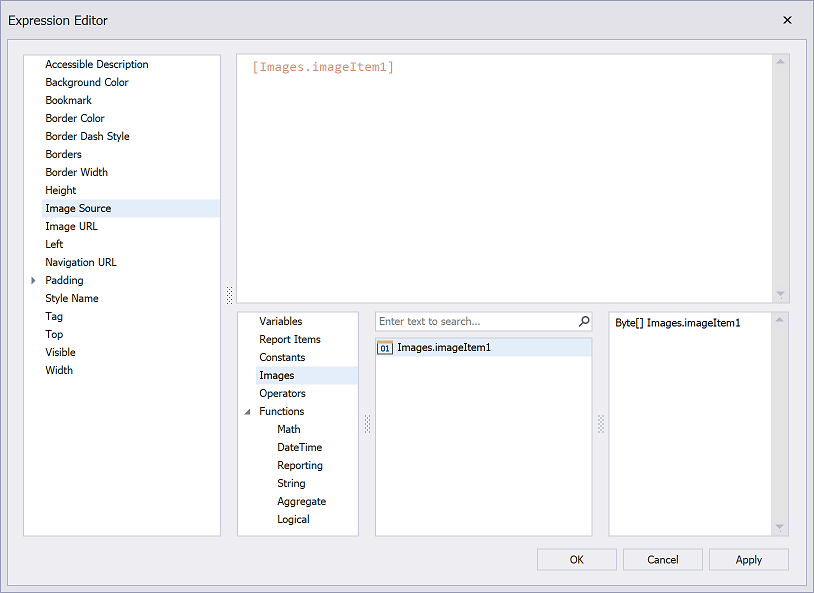# Expression Language

This section describes the report-specific expression syntax. Refer to the following topic in the Cross-Platform Core Libraries documentation for a list of basic constants, operators, and functions you can use in other DevExpress products: Criteria Language Syntax.

## Expression Syntax

An expression is a text string that specifies what data to take and how to process it to obtain a value. For instance, the following expression returns an integer value of `5`:

`3 + 2`

An expression string can consist of multiple lines that include constants, operators, function calls, fields or parameters, report items, and comments:

``````/*
This expression is set for the Visible property of a control
to show/hide the control based on the ShowTotalAmount parameter value.
*/

Iif (
?ShowTotalAmount == True,
True,
False
)
``````

## Constants

Constant

Description

Example

String constants

Wrap string constants in apostrophes.

If a string contains an apostrophe, double the apostrophe.

[Country] == ‘France’

[Name] == ‘O’’Neil’

Date-time constants

Wrap date-time constants in ‘#’.

[OrderDate] >= #2018-03-22 13:18:51.94944#

True

The Boolean True value.

[InStock] == True

False

The Boolean False value.

[InStock] == False

Enumeration

Specify an enumeration value by its underlying integer value.

[Status] == 1

You cannot specify an enumeration value by its qualified name. The following criterion is incorrect:

``````[Status] = Status.InProgress
``````

Call static methods of the EnumProcessingHelper class to register custom enumerations and refer to enumeration values as follows:

``````Status = ##Enum#MyNamespace.Status,InProgress#
``````

Guid

Wrap a Guid constant in curly braces. Use Guid constants in a relational operation with equality or inequality operators only.

[OrderID] == {513724e5-17b7-4ec6-abc4-0eae12c72c1f}

Numeric

Specify numeric constant types in a string form by suffixes:

• Int32 (int) - 1
• Int16 (short) - 1s
• Byte (byte) - 1b
• Double (double) - 1.0
• Single (float) - 1.0f
• Decimal (decimal) - 1.0m
 32-bit integer No suffix [CategoryID] == 1 16-bit integer s [CategoryID] == 1s Byte b [CategoryID] == 1b Double-precision floating-point number No suffix [Length] == 1.0 Single-precision floating-point number f [Length] == 1.0f Decimal floating-point number m [Price] == 25.0m

?

A null reference that does not refer to any object.

We recommend that you use the IsNull unary operator (for example, “[Region] is null”) or the IsNull logical function (for example, “IsNull([Region])”) instead of ?.

[Region] != ?

## Operators

Operator

Description

Example

+

Adds the value of one numeric expression to another or concatenates two strings.

[UnitPrice] + 4

[FirstName] + ‘ ‘ + [LastName]

-

Finds the difference between two operands.

[Price1] - [Price2]

*

Multiplies the value of two operands.

[Quantity] * [UnitPrice]

/

Divides the first operand by the second.
If both operands are integer, the resulting value is integer too. For example, `10/4` returns the value of `2`.
To return fractional values, ensure that at least one operand is of a floating-point type: `ToDecimal(10)/4` returns the value of `2.5`.

[Quantity] / 2

%

Divides one numeric operand by the other and returns the remainder (modulus).

[Quantity] % 3

|

Performs a bitwise inclusive OR operation on two numeric expressions. Compares each bit of its first operand to the corresponding bit of its second operand. If either bit is 1, the corresponding resulting bit is set to 1. Otherwise, the corresponding resulting bit is set to 0.

[Number] | [Number]

&

The bitwise AND operator. Compares each bit of its first operand to the corresponding bit of its second operand. If the two bits are 1, the corresponding resulting bit is set to 1. Otherwise, the corresponding resulting bit is set to 0.

[Number] & 10

^

Performs a bitwise exclusive OR operation on two numeric expressions.

[Number] ^ [Number]

==

=

Returns True if both operands are equal; otherwise, it returns False.

[Quantity] == 10

!=

Returns True if the operands are not equal; otherwise, it returns False.

[Country] != ‘France’

<

Less than operator. Used to compare expressions.

[UnitPrice] < 20

<=

Less than or equal to operator. Used to compare expressions.

[UnitPrice] <= 20

>=

Greater than or equal to operator. Used to compare expressions.

[UnitPrice] >= 30

>

Greater than operator. Used to compare expressions.

[UnitPrice] > 30

In (,,,)

Tests for the existence of a property in an object.

[Country] In (‘USA’, ‘UK’, ‘Italy’)

Between (,)

Specifies a range to test. Returns True if a value is greater than or equal to the first operand and less than or equal to the second operand.

[Quantity] Between (10, 20)

And

&&

Performs a logical conjunction on two Boolean expressions.

[InStock] And ([ExtendedPrice]> 100)

[InStock] && ([ExtendedPrice]> 100)

Or

||

Performs a logical disjunction on two Boolean expressions.

[Country]==’USA’ Or [Country]==’UK’

[Country]==’USA’ || [Country]==’UK’

~

Performs a bitwise negation on a numeric expression.

~[Roles] = 251

Not

!

Performs a logical negation on a Boolean expression.

Not [InStock]

![InStock]

+

Returns a numeric expression’s value (a unary operator).

+[Value] = 10

-

Returns the negative of a numeric expression’s value (a unary operator).

-[Value] = 20

Is Null

Returns True if an expression is a null reference (one that does not refer to any object).

[Region] is null

## Operator Precedence

When an expression contains multiple operators, these operators are evaluated in the following sequence:

• Literal values
• Parameters
• Identifiers
• OR (left-associative)
• AND (left-associative)
• The ‘.’ relationship qualifier (left-associative)
• ==, !=
• <, >, <=, >=
• -, + (left-associative)
• *, /, % (left-associative)
• NOT
• Unary -
• In
• Iif
• Trim(), Len(), Substring(), IsNull()
• ‘[]’ (for set-restriction)
• ‘()’

Group elements with parentheses to change operator precedence. For instance, operators are applied in the default order in the following expression:

`Accounts[Amount == 2 + 48 * 2]`

In the next expression, the addition operation is applied first, because its associated elements are grouped with parentheses, and the multiplication operation is applied last.

`Accounts[Amount == (2 + 48) * 2]`

## Functions

The expression language includes a set of functions that extend an expression’s capabilities:

• Logical functions
• Date and time functions
• Math functions
• String functions
• Functions for expression bindings and calculated fields
• Functions for stored procedures
• Functions for the Summary Expression Editor

You can also implement custom functions.

See the following topic for a complete list of functions that are available in expressions: Functions in Expressions.

## Case Sensitivity

Operators are case-insensitive. Case sensitivity of values can depend on the data source.

If SQL Server is configured as case-insensitive, the following filter expression always evaluates to `True`:

``````Lower(Name) == Upper(Name)
``````

## Escape Keywords

You can mark a keyword-like field name with the `@` escape character. In the expression below, the CriteriaOperator.Parse method interprets `@Or` as a field named Or, not the logical operator `OR`.

``````@Or = 'value'
``````

## Escape Characters

Use a backslash (`\`) as an escape character for characters in an expression, as shown below:

`\[`

`\\`

`\'`

Use an apostrophe (`'`) as an escape character for string literals:

``````'A parameter''s value is:' + ?parameter1
``````

## Data Fields and Calculated Fields

Enclose a data field or calculated field’s name in square brackets (`[` and `]`):

``````/*
This expression is set for a control's Text property
to bind the control to the UnitPrice data field.
*/
[UnitPrice]
``````

The report supports two notations to access fields in expressions:

Short Notation
`[FieldName]` - works in all expressions.
Long/Full Notation
`[TableName.FieldName]` - works only in binding expressions.

Note

Data field names are case insensitive.

Ensure that the field with the specified name exists in the report’s data source and data member.

You can refer to data fields from a data member that is not specified as the report’s data member (only the first record is returned):

``````/*
This expression is set for a control's Text property
to bind the control to the UnitPrice data field from the Products data member
(the report is not bound to Products).
*/
[Products].[UnitPrice]
``````

## Summary Expressions

Use the following format to construct a valid aggregate expression:

[<Collection>][<Condition>].<Aggregate>(<Expression>)

• <Collection> - Specifies a collection against which to calculate an aggregated value. It can be the relationship name for a master-detail relationship, or a collection property’s name exposed by the target class. For example, [CategoriesProducts][[CategoryId]>5].Count(). Empty brackets [] indicate the root collection.
• <Condition> - Specifies a condition that defines which records to use for the aggregate function calculation. To calculate an aggregated value against all records, delete this logical clause and its square brackets (for example, [].Count()).
• <Aggregate> - Specifies one of the available aggregate functions listed in the Aggregate enumeration.
• <Expression> - Specifies the expression to use (for example, [][[CategoryID] > 5].Sum([UnitPrice]*[Quantity])). The Count function does not require field values to count the records (the round brackets can be empty for this function).

Use the Parent Relationship Traversal Operator (‘^’) to refer to the processed group (for instance, [][[^.CategoryID] == [CategoryID]].Sum([UnitPrice])). This operator allows you to calculate aggregates within groups.

## Report Parameters

Use the following syntax to insert report parameters in an expression:

• Type a question mark before a parameter’s name.

`?parameter1`

• (Obsolete) Use the “Parameters.” prefix in front of a report parameter’s name.

`[Parameters.parameter1]`

## Enumerations

Do one of the following to assign an enumeration value to a property:

• Specify an enumeration value by its underlying integer value.

`[Borders] = 1`

• Call the EnumProcessingHelper.RegisterEnum static methods to register an enumeration at application startup:

``````DevExpress.Data.Filtering.EnumProcessingHelper.RegisterEnum(
typeof(DevExpress.XtraPrinting.BorderSide));
``````

After that, you can refer to enumeration values as follows:

`[Borders] = ##Enum#DevExpress.XtraPrinting.BorderSide,Left#`

• The Expression Editor can help you specify a string value for built-in enumerations:## Collections

[Images]

Allows you to reference the images stored in the report’s ImageResources collection.

The following expression binds a PictureBox control’s ImageSource property to an element from the ImageResources collection:

`[Images.imageItem1]```````/*
Comments start with the `/*` sequence and end at the matching `*/` sequence.